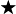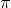HELP ME PLSSSS!!!!! If the diameter of the circle above is 16 mm, what is the area of the circle? A. 16 mm2 B.

Question

HELP ME PLSSSS!!!!!
If the diameter of the circle above is 16 mm, what is the area of the circle?
A.
16 mm2
B.
64 mm2
C.
8 mm2
D.
256 mm2

in progress 0
5 months 2021-08-26T19:47:43+00:00 2 Answers 0 views 0

1. Question:-

If the diameter of the circle above is 16 mm, what is the area of the circle ?

Solution:-

Diameter of the circle = 16 mm. (Given)

Radius (r) of the circle = 16/2 = 8 mm.To Find the area of the circle.

Since we know,Area of circle is πr².

So, Area of the circle = 3.14 × (8)²

Area of the circle = 3.14 × 8 × 8

Area of the circle = 200.96 = 201 mm².

The area of the circle is 201mm². [Answer]

64mm² or approximately 201.06 mm²

Step-by-step explanation:

If the diameter is 16 mm, the radius will be 8 mm.

Use the circle area formula, A =r², where r is the radius. Plug in 8 as r:

A =A =(8²)

A = 64So, the area of the circle is 64mm² or approximately 201.06 mm²